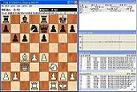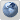WBEC-Ridderkerk forum
Would you like to react to this message? Create an account in a few clicks or log in to continue.

# WBEC Ridderkerk, 3rd Div final test result..##WBEC Ridderkerk, 3rd Div final test result..`Test at Dual Opteron 244 at 40/5, ponder=onOPTERON-A, 2009.05.20 - 2009.05.21                       Score     D M P C Q L B T R C D G--------------------------------------------------------- 1: Delphil 1.9g      9.0 / 11   X 1 1 = 1 1 1 1 1 0 = 1 2: Muse 0.899b uci   7.0 / 11   0 X 1 1 0 0 1 0 1 1 1 1 3: Pupsi2 0.07       7.0 / 11   0 0 X 1 1 0 = 1 1 1 1 = 4: Capture R01       6.0 / 11   = 0 0 X = = 1 1 1 0 = 1 5: Queen 4.03        6.0 / 11   0 1 0 = X 1 = = 0 1 1 = 6: Leila 0.53h       5.0 / 11   0 1 1 = 0 X 0 0 1 1 = 0 7: Bruja 1.9         5.0 / 11   0 0 = 0 = 1 X = = 1 0 1 8: Tytan 9.32-x64    4.5 / 11   0 1 0 0 = 1 = X 0 0 1 = 9: Resp 0.19-x64     4.5 / 11   0 0 0 0 1 0 = 1 X 1 0 110: Chezzz 1.0.3      4.0 / 11   1 0 0 1 0 0 0 1 0 X = =11: Diablo 0.5.1      4.0 / 11   = 0 0 = 0 = 1 0 1 = X 012: Gaia 3.5          4.0 / 11   0 0 = 0 = 1 0 = 0 = 1 X---------------------------------------------------------66 games: +30 =16 -20`

Problems found:

LGpgnver:
0 warnings/errors found

Best wishes,
Leo.

Games of this testLeo Dijksman

Number of posts : 454
Age : 70
Location : Stavenisse
Registration date : 2008-05-17Permissions in this forum:
You cannot reply to topics in this forum